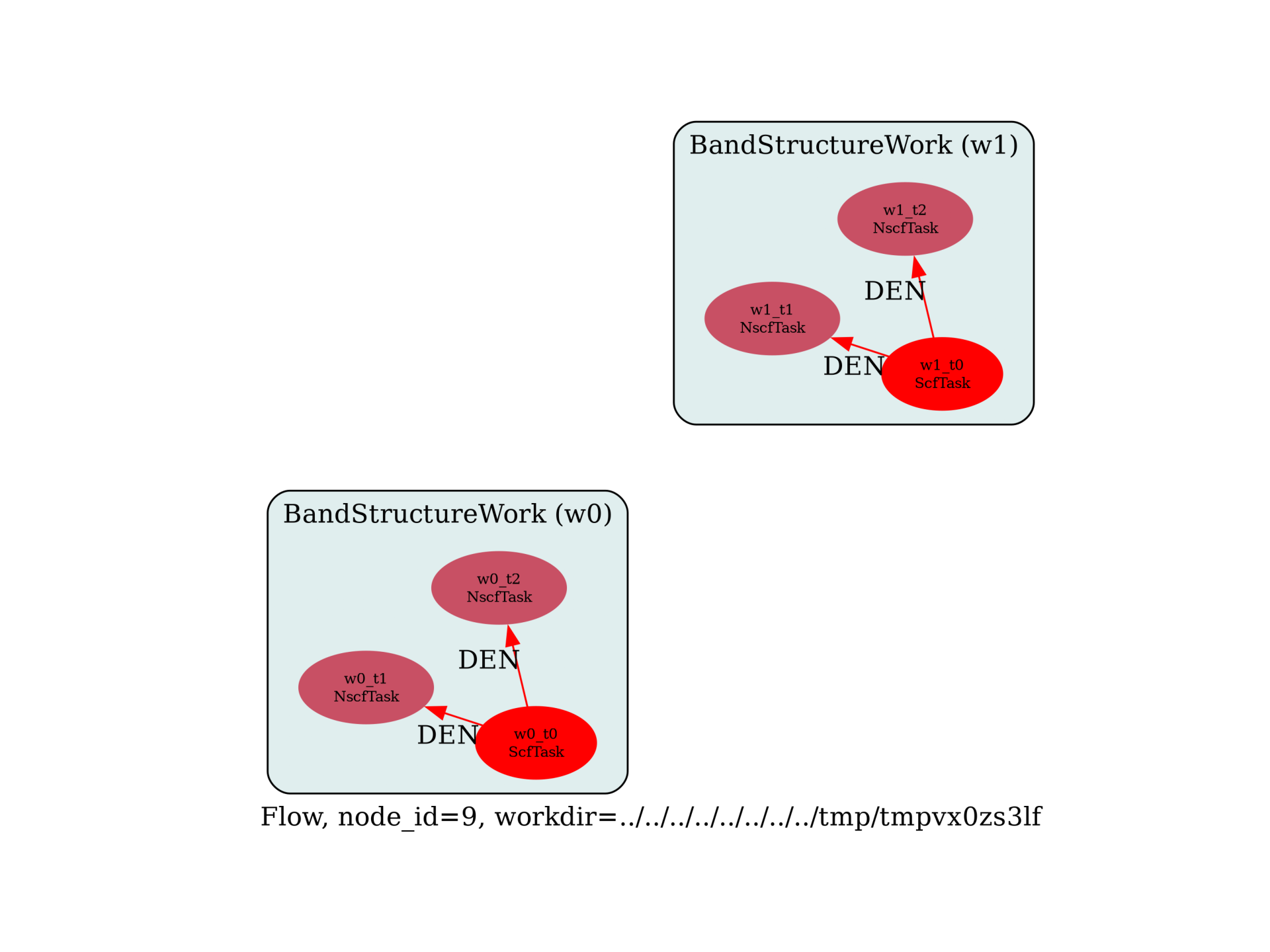Band structure w/wo magnetization¶

Calculation of the band structure of Fe with and without magnetization. See also <~abinit/tutorial/Input/tspin_1.in>

import os
import sys
import abipy.data as data
import abipy.abilab as abilab
import abipy.flowtk as flowtk

def make_scf_nscf_inputs(nsppol, paral_kgb=1):
"""
Generate two input files for the GS and the NSCF run for given `nsppol`.
"""
# Fe normal bcc structure for test of a ferromagnetic calculation
multi = abilab.MultiDataset(structure=data.structure_from_ucell("Fe-fm"),
pseudos=data.pseudos("26fe.pspnc"), ndtset=2)

# Global variables
global_vars = dict(
nsppol=nsppol,
ecut=18,
nband=8,
occopt=3,
tsmear=0.01,
paral_kgb=paral_kgb,
)
if nsppol == 2:
global_vars.update(spinat=[0.0, 0.0, 4.0])

multi.set_vars(global_vars)

# Dataset 1 (GS run)
multi.set_kmesh(ngkpt=[4,4,4], shiftk=[0.5,0.5,0.5])
multi.set_vars(tolvrs=1e-6)

# Dataset 2 (NSCF run)
multi.set_kpath(ndivsm=4)
multi.set_vars(tolwfr=1e-8)

# Generate two input files for the GS and the NSCF run
scf_input, nscf_input = multi.split_datasets()

return scf_input, nscf_input

def build_flow(options):
# Working directory (default is the name of the script with '.py' removed and "run_" replaced by "flow_")
if not options.workdir:
options.workdir = os.path.basename(sys.argv).replace(".py", "").replace("run_", "flow_")

# Create the Flow.
flow = flowtk.Flow(options.workdir, manager=options.manager)

# Create the task defining the calculation and run and register it in the flow
for nsppol in [1, 2]:
scf_input, nscf_input = make_scf_nscf_inputs(nsppol)
work = flowtk.BandStructureWork(scf_input, nscf_input)
flow.register_work(work)

return flow

# This block generates the thumbnails in the AbiPy gallery.
# You can safely REMOVE this part if you are using this script for production runs.
__name__ = None
import tempfile
options = flowtk.build_flow_main_parser().parse_args(["-w", tempfile.mkdtemp()])
build_flow(options).graphviz_imshow()

@flowtk.flow_main
def main(options):
"""
This is our main function that will be invoked by the script.
flow_main is a decorator implementing the command line interface.
Command line args are stored in `options`.
"""
return build_flow(options)

if __name__ == "__main__":
sys.exit(main())Run the script with:

run_fe_ebands -s

then use:

abirun.py flow_fe_ebands ebands -p

to analyze all the band structures produced by the Flow and plot the dataTotal running time of the script: ( 0 minutes 0.655 seconds)

Gallery generated by Sphinx-Gallery